A depth camera measures the distance from the camera to a point in 3D space. For a given point, the camera supplies the row and column on its ‘screen’ and the depth towards the point. It is worth pointing out here that classic depth cameras like the Kinect supply the length of the ray; RealSense cameras supply the range, or Z component.

Calculating the coordinates of the point is fairly straightforward trigonometry. Suppose a D435 camera is mounted 500mm off the ground, pointing at the horizon. 1000mm away there is an object 101.5mm high: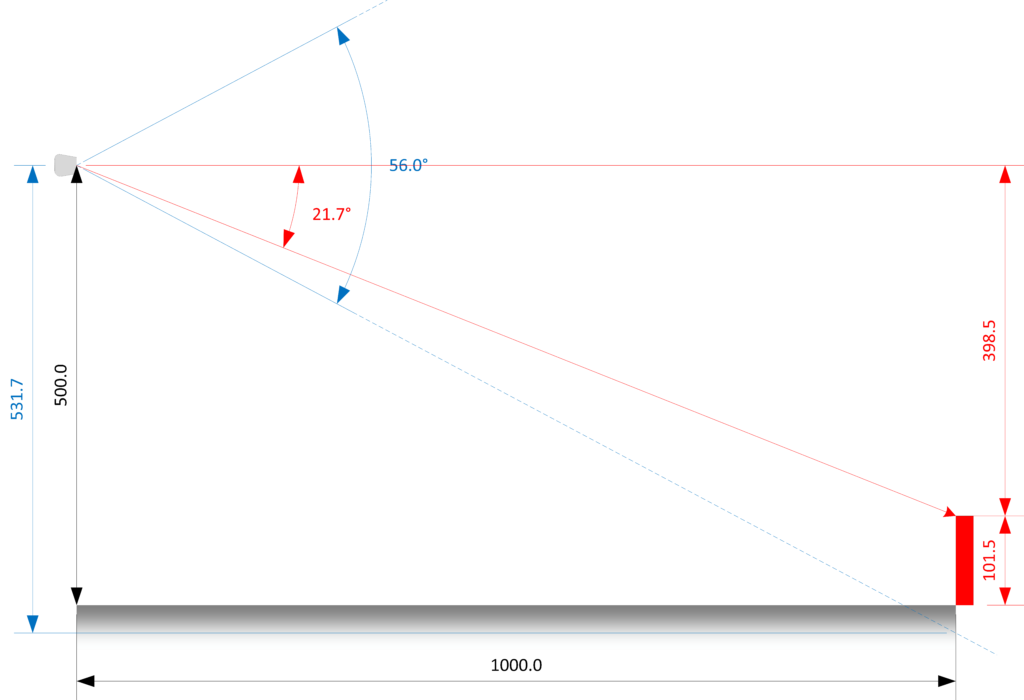To warm up, the camera’s vertical field of view is 56°, so at 1’000mm half of the height is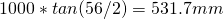The camera has 480 rows, so it will see the 101.5mm-high object at row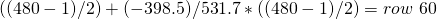Bonus: It sees the object at an angle of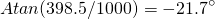Now we define constants for the Fx intrinsics, the centre row and the height of a pixel: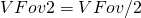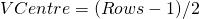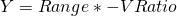Notice the ‘Rows-1’ because there are 479 intervals between 480 pixels: row 239 points just under the horizon and row 240 points just above the horizon.

Then, for the example above we define our constants: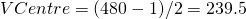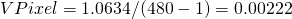and calculate the Y coordinate: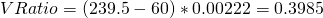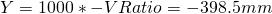The calculations for the X-coordinate are identical, replacing ‘Vertical’ with ‘Horizontal’ and Z is simply the supplied range.